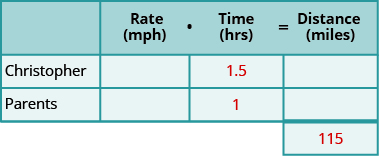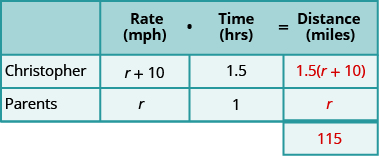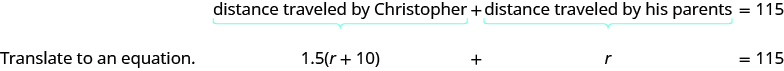# 3.5 Solve uniform motion applications  (Page 2/7)

 Page 2 / 7

Wayne and Dennis like to ride the bike path from Riverside Park to the beach. Dennis’s speed is seven miles per hour faster than Wayne’s speed, so it takes Wayne 2 hours to ride to the beach while it takes Dennis 1.5 hours for the ride. Find the speed of both bikers.

Wayne 21 mph, Dennis 28 mph

Jeromy can drive from his house in Cleveland to his college in Chicago in 4.5 hours. It takes his mother 6 hours to make the same drive. Jeromy drives 20 miles per hour faster than his mother. Find Jeromy’s speed and his mother’s speed.

Jeromy 80 mph, mother 60 mph

In [link] , the last example, we had two trains traveling the same distance. The diagram and the chart helped us write the equation we solved. Let’s see how this works in another case.

Christopher and his parents live 115 miles apart. They met at a restaurant between their homes to celebrate his mother’s birthday. Christopher drove 1.5 hours while his parents drove 1 hour to get to the restaurant. Christopher’s average speed was 10 miles per hour faster than his parents’ average speed. What were the average speeds of Christopher and of his parents as they drove to the restaurant?

## Solution

Step 1. Read the problem. Make sure all the words and ideas are understood.

• Draw a diagram to illustrate what it happening. Below shows a sketch of what is happening in the example.• Create a table to organize the information.
• Label the columns rate, time, distance.
• List the two scenarios.
• Write in the information you know.Step 2. Identify what we are looking for.

• We are asked to find the average speeds of Christopher and his parents.

Step 3. Name what we are looking for. Choose a variable to represent that quantity.

• Complete the chart.
• Use variable expressions to represent that quantity in each row.
• We are looking for their average speeds. Let’s let r represent the average speed of the parents. Since the Christopher’s speed is 10 mph faster, we represent that as $r+10.$

Fill in the speeds into the chart.Multiply the rate times the time to get the distance.

Step 4. Translate into an equation.

• Restate the problem in one sentence with all the important information.
• Then, translate the sentence into an equation.
• Again, we need to identify a relationship between the distances in order to write an equation. Look at the diagram we created above and notice the relationship between the distance Christopher traveled and the distance his parents traveled.

The distance Christopher travelled plus the distance his parents travel must add up to 115 miles. So we write:Step 5. Solve the equation using good algebra techniques.

$\begin{array}{cccc}\text{Now solve this equation.}\hfill & & & \hfill \begin{array}{ccc}\hfill 1.5\left(r+10\right)+r& =\hfill & 115\hfill \\ \hfill 1.5r+15+r& =\hfill & 115\hfill \\ \hfill 2.5r+15& =\hfill & 115\hfill \\ \hfill 2.5r& =\hfill & 100\hfill \\ \hfill r& =\hfill & 40\hfill \end{array}\hfill \\ & & & \text{So the parents’ speed was 40 mph.}\hfill \\ \text{Christopher’s speed is}\phantom{\rule{0.2em}{0ex}}r+10.\hfill & & & \hfill \begin{array}{c}\hfill r+10\hfill \\ \hfill 40+10\hfill \\ \hfill 50\hfill \end{array}\hfill \\ & & & \text{Christopher’s speed was 50 mph.}\hfill \end{array}$

Step 6. Check the answer in the problem and make sure it makes sense.

$\begin{array}{cccccc}\phantom{\rule{2.7em}{0ex}}\text{Christopher drove}\hfill & & & \text{50 mph (1.5 hours)}\hfill & =\hfill & \text{75 miles}\hfill \\ \phantom{\rule{2.7em}{0ex}}\text{His parents drove}\hfill & & & \text{40 mph (1 hours)}\hfill & =\hfill & \underset{\text{_______}}{\text{40 miles}}\hfill \\ & & & & & \text{115 miles}\hfill \end{array}$

$\begin{array}{cccc}\begin{array}{c}\mathbf{\text{Step 7. Answer}}\phantom{\rule{0.2em}{0ex}}\text{the question with a complete sentence.}\hfill \\ \\ \end{array}\hfill & & & \begin{array}{c}\text{Christopher’s speed was 50 mph.}\hfill \\ \text{His parents’ speed was 40 mph.}\hfill \end{array}\hfill \end{array}$

rectangular field solutions
What is this?
Donna
the proudact of 3x^3-5×^2+3 and 2x^2+5x-4 in z7[x]/ is
?
Choli
a rock is thrown directly upward with an initial velocity of 96feet per second from a cliff 190 feet above a beach. The hight of tha rock above the beach after t second is given by the equation h=_16t^2+96t+190
Usman
Stella bought a dinette set on sale for $725. The original price was$1,299. To the nearest tenth of a percent, what was the rate of discount?
44.19%
Scott
40.22%
Terence
44.2%
Orlando
I don't know
Donna
if you want the discounted price subtract $725 from$1299. then divide the answer by $1299. you get 0.4419... but as percent you get 44.19... but to the nearest tenth... round .19 to .2 and you get 44.2% Orlando you could also just divide$725/$1299 and then subtract it from 1. then you get the same answer. Orlando p mulripied-5 and add 30 to it Tausif Reply p mulripied-5 and add30 Tausif p mulripied-5 and addto30 Tausif Can you explain further Monica Reply p mulripied-5 and add to 30 Tausif How do you find divisible numbers without a calculator? Jacob Reply TAKE OFF THE LAST DIGIT AND MULTIPLY IT 9. SUBTRACT IT THE DIGITS YOU HAVE LEFT. IF THE ANSWER DIVIDES BY 13(OR IS ZERO), THEN YOUR ORIGINAL NUMBER WILL ALSO DIVIDE BY 13!IS DIVISIBLE BY 13 BAINAMA When she graduates college, Linda will owe$43,000 in student loans. The interest rate on the federal loans is 4.5% and the rate on the private bank loans is 2%. The total interest she owes for one year was $1,585. What is the amount of each loan? Ariana Reply Sean took the bus from Seattle to Boise, a distance of 506 miles. If the trip took 7 2/3 hours, what was the speed of the bus? Kirisma Reply 66miles/hour snigdha How did you work it out? Esther s=mi/hr 2/3~0.67 s=506mi/7.67hr = ~66 mi/hr Orlando hello, I have algebra phobia. Subtracting negative numbers always seem to get me confused. Alicia Reply what do you need help in? Felix subtracting a negative....is adding!! Heather look at the numbers if they have different signs, it's like subtracting....but you keep the sign of the largest number... Felix for example.... -19 + 7.... different signs...subtract.... 12 keep the sign of the "largest" number 19 is bigger than 7.... 19 has the negative sign... Therefore, -12 is your answer... Felix —12 Niazmohammad Thanks Felix.l also get confused with signs. Esther Thank you for this Shatey ty Graham think about it like you lost$19 (-19), then found $7(+7). Totally you lost just$12 (-12)
Annushka
I used to struggle a lot with negative numbers and math in general what I typically do is look at it in terms of money I have -$5 in my account I then take out 5 more dollars how much do I have in my account well-$10 ... I also for a long time would draw it out on a number line to visualize it
Meg
practicing with smaller numbers to understand then working with larger numbers helps too and the song/rhyme same sign add and keep opposite signs subtract keep the sign of the bigger # then you'll be exact
Meg
Bruce drives his car for his job. The equation R=0.575m+42 models the relation between the amount in dollars, R, that he is reimbursed and the number of miles, m, he drives in one day. Find the amount Bruce is reimbursed on a day when he drives 220 miles
168.50=R
Heather
john is 5years older than wanjiru.the sum of their years is27years.what is the age of each
46
mustee
j 17 w 11
Joseph
john is 16. wanjiru is 11.
Felix
27-5=22 22÷2=11 11+5=16
Joyce
I don't see where the answers are.
Ed
Cindy and Richard leave their dorm in Charleston at the same time. Cindy rides her bicycle north at a speed of 18 miles per hour. Richard rides his bicycle south at a speed of 14 miles per hour. How long will it take them to be 96 miles apart?
3
Christopher
18t+14t=96 32t=96 32/96 3
Christopher
show that a^n-b^2n is divisible by a-b
What does 3 times your weight right now
Use algebra to combine 39×5 and the half sum of travel of 59+30
Cherokee
What is the segment of 13? Explain
Cherokee
my weight is 49. So 3 times is 147
Cherokee
kg to lbs you goin to convert 2.2 or one if the same unit your going to time your body weight by 3. example if my body weight is 210lb. what would be my weight if I was 3 times as much in kg. that's you do 210 x3 = 630lb. then 630 x 2.2= .... hope this helps
tyler
How to convert grams to pounds?
paulBy RhodesBy Megan EarhartBy Lakeima RobertsBy OpenStaxBy Yasser IbrahimBy OpenStaxBy OpenStaxBy JavaChamp TeamBy Dan ArielyBy OpenStax